### What are your chances of acceptance?

Duke University
UCLA
Unweighted GPA: 3.7
1.0
4.0
SAT: 720 math
200
800
| 800 verbal
200
800

#### Extracurriculars

Low accuracy (4 of 18 factors)Nisha Desai
10

# 15 Hard ACT Science Questions to Improve Your Score

### Do you know how to improve your profile for college applications?

See how your profile ranks among thousands of other students using CollegeVine. Calculate your chances at your dream schools and learn what areas you need to improve right now — it only takes 3 minutes and it's 100% free.

## What’s Covered:

The ACT Science Test is the last of four sections on the ACT. For many students, it’s the most intimidating, as they’re not sure how to approach the charts and data sets.

In this post, we’ll cover 15 of the hardest ACT Science questions, with corresponding explanations of how to answer them.

## Overview of the ACT Science Test

The ACT science test requires you to complete 40 questions in 35 minutes. These questions test your interpretation, analysis, evaluation, reasoning, and problem-solving skills. You should know general information about biology, chemistry, physics, and the Earth/space sciences, but advanced knowledge of any of these subjects isn’t required. The format of the test involves seven brief passages, accompanied by five to seven multiple-choice questions.

There are three different categories of questions that you’ll be asked:

1. Interpretation of Data (45–55%)

These questions require you to manipulate and analyze data presented in tables, graphs, and diagrams.

2. Scientific Investigation (20–30%)

This type of question involves understanding experimental tools, procedures, and design.

3. Evaluation of Models, Inferences, and Experimental Results (25–35%)

These questions test your ability to judge the validity of scientific information and then formulate conclusions and predictions.

## How Will the ACT Affect My College Chances?

Most colleges heavily rely on standardized testing as an indicator of a student’s academic success. Some selective schools even filter out applicants with low ACT or SAT scores, though this has changed recently in light of the COVID-19 pandemic. Still, a strong score can certainly give your application a boost, even with test-optional policies.

To see how your ACT score affects your college chances, use CollegeVine’s free Admissions Calculator. This tool will not only predict your admissions chances at schools, but also highlight what other aspects of your profile can be improvedlike extracurriculars, grades, and more!## 15 Hardest ACT Science Questions

Here are fifteen examples of more difficult types of questions that you may encounter on the ACT Science Test.

### Passage I, Question 1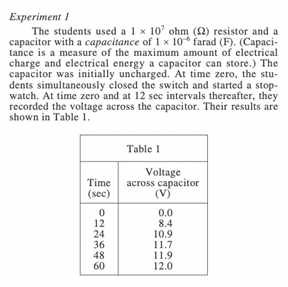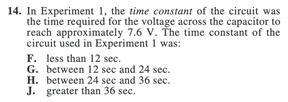Since the question only asks about Experiment 1, look at Table 1. Looking at other tables may confuse you or lead you to the wrong answer, so if the question directs you towards a particular experiment, try to stay focused on those graphics only.

Since the voltage we’re discussing is 7.6 V, we need to look for values close to this on the table. 7.6 falls in between the values 0.0 and 8.4, and the times associated with those voltages are 0.0 and 12, respectively.

So, the correct answer should be less than 12 seconds.

### Passage I, Question 2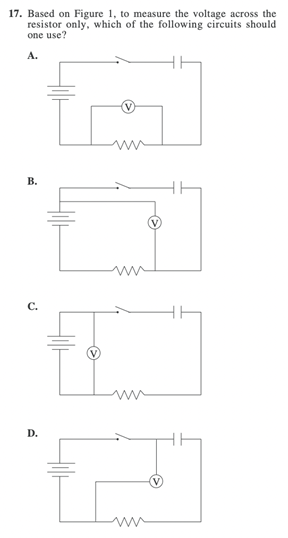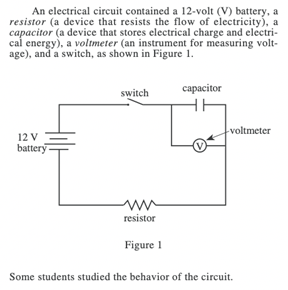This question definitely requires knowledge of circuits, which makes it more difficult, since most students don’t encounter circuits in high school (unless you’ve taken a more advanced physics course).

Since the switch is open in each of these circuits, the voltmeter will only measure the path which is closed (i.e. the loop which doesn’t contain the switch). For example, in answer choice C, the voltmeter only measures the batterythe resistor and capacitor are within the path that contains the switch, so they won’t affect the voltmeter reading.

In answer choice D, the resistor is correctly included, but the capacitor is also included, so this is also incorrect.

Answer choice B is similarly incorrect in that the resistor and the battery are being measured.

So, answer choice A is correct, since the closed path only contains the resistor.

### Passage I, Question 3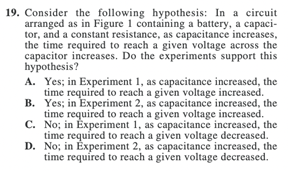The hypothesis discusses a relationship between capacitance and time. So, to narrow our focus, let’s look at Experiment 2, since Table 2 relates these two variables. In Table 2, we see that as capacitance increases, time also increases. So, the correct answer is B. Again, with this question, it’s important to recognize which information from the passage is necessary to obtain the correct answer.

### Passage II, Question 1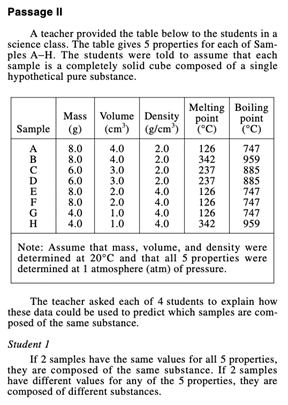This passage involves student explanations, which can be tricky, since they aren’t necessarily correct. Questions corresponding to these passages don’t really ask you to judge the validity of student explanations, but rather, to make predictions or assumptions on their behalf, based on your assessment of their thinking.

When approaching these types of problems, try jotting down a short description of the student’s explanation. This will allow you to answer the questions much faster. For this problem specifically, we’re only looking at Student 3. Their explanation can be briefly summarized as follows “same substances have the same mass, volume, AND density.”

Based on their explanation, let’s look for which substances have all three of these values equivalent. From the table, samples A and B, C and D, E and F, and G and H would be the same substances– be careful to avoid looking at the melting/boiling points when analyzing the table, since Student 3 said those properties were not relevant.

Since G and H is the only answer choice that matches one of the pairs in our list, the correct answer choice is J.

### Passage II, Question 2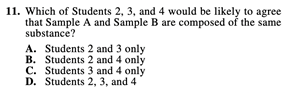For this question, we’ll need to look at multiple students. To make this process faster, let’s briefly summarize each student’s descriptions of what two substances need to be the same:

Student 2: 3 or more of the same values (it doesn’t matter which 3)

Student 3: the same mass, volume, and density

Student 4: the same density, melting point, and boiling point

Now, let’s analyze samples A and B:

Mass: same

Volume: same

Density: same

Melting point: different

Boiling point: different

Because at least three properties have the same values, student 2 would agree.

Because the mass, volume, and density are the same, student 3 would agree.

Because the melting and boiling points are different, student 4 would not agree.

So, the correct answer is A.

### Passage II, Question 3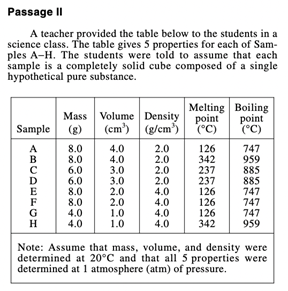This question is particularly difficult in that it requires you to have a rudimentary understanding of phases of matter and the meaning of melting/boiling points.

The question is asking for Sample D’s density at 890°C. From the table, we can see that this value is above sample D’s boiling point (which is 885°C). So, we need to use prior knowledge that once a substance is past the boiling point, it is in the gas phase. Now, we can eliminate answer choices B and D, since they incorrectly state that sample D is a liquid.

Next, we’ll need to again use prior knowledge. The ACT expects you to know that gases generally have lower densities than solids. This will allow you to correctly choose A as the answer.

### Passage III, Question 1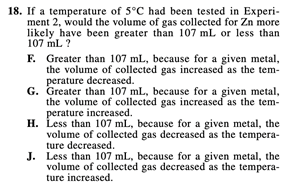This question is tricky since the answer cannot be explicitly found in the given graphs. Rather, it requires additional analysis to extrapolate the data. This means we’ll have to use the trend shown in the graph to estimate data values for points beyond the range shown in the graph. Since the question asks about Experiment 2, we should look at Figure 2.

The question is asking for the volume of gas collected for Zn when the temperature is 5°C. Since the graph doesn’t have data for this temperature, we’ll have to make an assumption. The slope of the line corresponding to Zn is positive, volume and temperature both increase and decrease together. This means that a temperature lower than 10°C will likely correspond to a volume lower than 107 mL (which is the volume corresponding to 10°C).

At this point, we know the answer is either H or J, so we need to determine the reasoning to figure out the correct answer. Answer choice J is incorrect since it falsely states that volume decreases as temperature increases–yet, we can see in the graph that volume decreases when temperature also decreases. So, the correct answer is H.

### Passage III, Question 2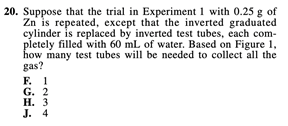Like the question before, this question’s difficulty comes from the fact that the answer is not explicitly shown in the data. First, since the question asks about Experiment 1, we need to look at Figure 1. We need to find out the number of 60 mL test tubes necessary to collect the gas produced from 0.25 g of Zn.

The first step is to figure out how much gas will need to be collected. From Figure 1, the volume corresponding to 0.25 g Zn is about 92. So, to find out the number of test tubes needed, let’s divide: $$92/60\:\approx\:1.53$$.

Since the answers are given in whole numbers, we now need to figure out whether to round down or up. If we rounded down to 1 test tube, there would be about 30 mL of gas that would remain uncollected. So, we should round our number up to 2 test tubes, to ensure that all the gas will be collected, making G the correct answer.

### Passage III, Question 3This question is more difficult since it requires some outside knowledge. You’ll need a basic understanding of balanced chemical equations to answer this problem. Let’s recall the given equation:Here, we see that for every 2 moles of $$HCl$$ consumed, 1 mole of $$H_2$$ is produced. Then, when 10 moles of $$HCl$$ are consumed, we should have half that amount, or 5 moles, of $$H_2$$ produced.

If you know what the coefficients in a balanced chemical equation signify, this question should be fairly easy. So, the ACT expects you to understand balanced chemical equations so that you can extract information from them. Make sure to brush up on your chemistry knowledge while studying for the ACT!

## Discover your chances at hundreds of schools

Our free chancing engine takes into account your history, background, test scores, and extracurricular activities to show you your real chances of admission—and how to improve them.

### Passage IV, Question 1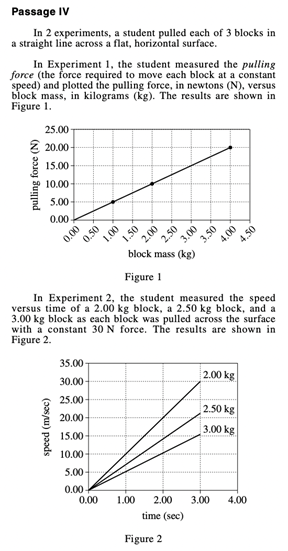Again, answering this question means you must rely on prior knowledge. In this case, you should know the relationship between acceleration and speed: acceleration is the rate of change of speed.

Since we’re given a graph of a line, another way to think of rate of change is the slope! Neither of the graphs mention acceleration specifically, so we’ll have to do this extra analysis. Since Figure 2 relates speed to time, finding the slope of the line will give us the rate of change of speed, which, contextually, is the acceleration. Be careful when looking at the graph, since we should be looking at the line associated with the 3.00kg block.

To find the slope, let’s pick two arbitrary points. If you can, picking the end points is an easy way to find the answer and involves the least amount of error. First, let’s pick $$(0,0)$$, since when the time is 0 seconds, the speed is 0 m/s. Next, let’s pick $$(3,15)$$, since the speed is 15 m/s when the time is 3 seconds.

Let’s use the slope formula:

$$m=\frac{15-0}{3-0}=\frac{15}{3}=5 m/s^2$$

So, the correct answer is B.

### Passage IV, Question 2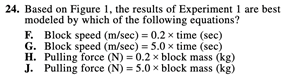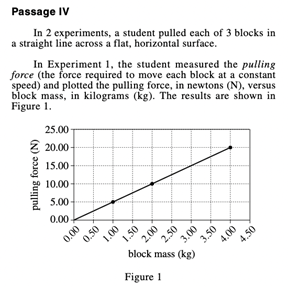This question is asking us to find the equation of the line in Figure 1. Immediately, we can eliminate answer choices F and G, since Figure 1 doesn’t show speed or time. (This is why reading the labels of graphs is very important!)

The trickier part is determining the relationship between the pulling force and the block mass. We can do this by thinking of it as the equation of a line.

In this case, our y is pulling force and our x is block mass. A typical equation of a line is $$y=mx$$. We don’t have the term “+b” since our y-intercept in this case is just 0.

So, to answer this question, we’ll need to determine m. Like before, we can pick two points to do so: $$(0,0)$$ and $$(4,20)$$. Make sure you use the slope formula correctly, as mixing up $$x$$ and $$y$$ will cause you to answer this problem incorrectly.

$$m=\frac{20-0}{4-0}=5.$$

Thus, the answer to this question is J.

### Passage IV, Question 3Once again, this question involves extrapolating data. This time, however, the question asks for a specific value. Though there are several ways to approach this problem, a convenient trick is to use your answer from the previous question.

We already determined that the relationship between force (F) and mass (m) was: $$F=5m$$.

Plug in 30:

$$30=5m$$

$$m=6$$.

After selecting this answer, to check your work, you should plot the point $$(6, 30)$$ and see if it connects to the existing line. This will not only confirm your work for this question, but also the previous question as well!

### Passage V, Question 1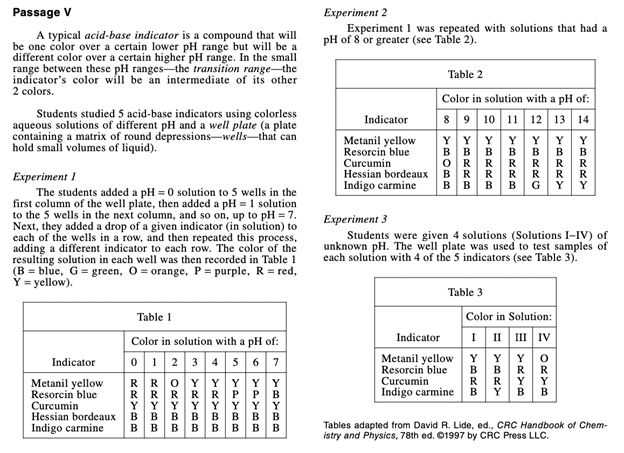This question involves comparing data from two separate tables. For curcumin, we see that it remains yellow for solutions with pH 1–7. Based on Table 2, curcumin turned orange for a solution with pH 8, then remained red for solutions with pH 9–14.

Reading the passage is necessary for this problem, especially the part that defines the transition range to be when the solution’s color is “an intermediate of its other 2 colors.”

For curcumin, the two colors are yellow and red, and the intermediate is orange. This intermediate occurs in a solution with pH 8, so the transition range should include 8.0, and C is the only answer choice which does this.

### Passage V, Question 2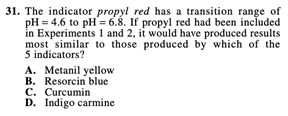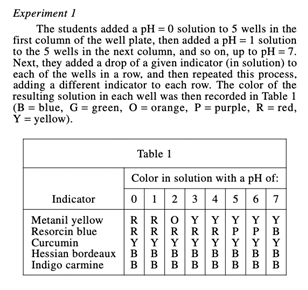The new information in this question might seem overwhelming, but really it’s only asking which of the 5 indicators in the passage would have a transition range of pH = 4.6 to pH = 6.8. Being able to determine the important information in the problem and discard all extraneous details is an important skill for the ACT Science section.

A transition range of pH = 4.6 to pH = 6.8 indicates the intermediate color will occur at either pH = 5 or 6 (since those are the only two whole numbers within this range). If we look at Tables 1 and 2, we see that resorcin blue fits this description, with its intermediate color (purple) occurring at pH 5 and 6.

### Passage V, Question 3This question involves comparing the student’s analysis to the information given in all of the tables. If student 3’s claim (that solution III has a pH of 7.3) is true, the colors should match the entries in Table 3.

Let’s compare the actual colors of each substance at a pH of 7 (from Table 1) to the colors of each substance in Solution III (from Table 3).

 Solution with pH of 7 Solution III Metanil yellow Y Y Resorcinol blue B R Curcumin Y Y Indigo carmine B B

If student 3’s claim is correct, these two columns should be identical. But, we see that resorcinol blue differs. Therefore, the correct answer is G.

## Final Tips

As you take the exam, make sure to read the passages very carefully. Often, small details will be necessary to answer certain questions. Also, for any figure, pay close attention to the description above or below it. These descriptions will help contextualize the graph and allow you to better understand the information given. If you encounter a question and you’re not sure what it’s asking, go back and reread the passage, just in case you misunderstood the information.

Remember, there is no penalty for incorrect answers, so it’s better to guess than to leave a question blank. So, if you find yourself running out of time, try to take the last minute or two to make sure your answer sheet is completely filled out.

Finally, the best way to improve your ACT Science score is to practice, practice, practice! Doing as many practice problems as you can will get you better accustomed to the format of the test and the types of questions. This will allow you to get through this section faster and with more accuracy.

10 Tips for Acing the ACT Science Section

15 Hardest ACT Math Questions to Improve Your Score